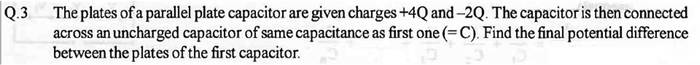# Capacitance question

## Homework Statement## The Attempt at a Solution

I don't have any idea on this.
Firstly, how are the capacitors connected? are they in series or parallel?
What happens to the charge on plates of first capacitor when the capacitors are connected in parallel?

Any help is appreciated. :)

## Answers and Replies

ehild
Homework Helper
The capacitors connected means that both plates of the first one are connected to the plates of the other one.

Note that charges can go from one plate to the other if the plates are connected.

At the end, the connected plates are at the same potential.

ehild

The capacitors connected means that both plates of the first one are connected to the plates of the other one.

Note that charges can go from one plate to the other if the plates are connected.

At the end, the connected plates are at the same potential.

ehild

So that means they are connected in parallel, right?

Here are different charges on both the plates of first capacitor, so i don't understand how the charge will flow, i mean how much charge will the second capacitor gain or lose?ehild
Homework Helper
Assuming the capacitors are equivalent, can you see any difference between them at the end?
You get a single capacitor, with twice as big plates as the original ones.
Find out the charge distribution. Are there charges only on the inside of the capacitor? Or are charges also on the outer surfaces of the plates?

ehild

Assuming the capacitors are equivalent, can you see any difference between them at the end?
You get a single capacitor, with twice as big plates as the original ones.
Find out the charge distribution. Are there charges only on the inside of the capacitor? Or are charges also on the outer surfaces of the plates?

ehild

Charges will be on both the surfaces.
Increasing the area will increase the capacity, but how can i find the charge distribution?

You said that they will at the same potential at the end. So can i use the conservation of charge method? If yes, how will i find the initial charge?

ehild
Homework Helper
The resultant capacitor has got 4Q charge on one plate and -2Q charge on the other plate. The size of the plates is twice the original area. The charge distribution should be even at the end.
The whole system has got 2Q excess charge. That excess charge distributes evenly on the outer surfaces. The potential difference between the plates is obtained from the charge of the inner surfaces.

ehild

The resultant capacitor has got 4Q charge on one plate and -2Q charge on the other plate. The size of the plates is twice the original area. The charge distribution should be even at the end.
The whole system has got 2Q excess charge. That excess charge distributes evenly on the outer surfaces. The potential difference between the plates is obtained from the charge of the inner surfaces.

ehild

Can we get back to the original situation when the area is not doubled because i am getting confused, please?
So the charge on the outer surfaces of the first capacitor is Q and on the inner surfaces is 3Q and -3Q, am i right?

ehild
Homework Helper
Can we get back to the original situation when the area is not doubled because i am getting confused, please?
So the charge on the outer surfaces of the first capacitor is Q and on the inner surfaces is 3Q and -3Q, am i right?

Yes.

ehild

Yes.

ehild

Thanks! So my next step would be equating final and initial charges?

3Q=CV+CV
(V is the common potential at the end)
From here i get V=3Q/2C.

Is my answer right?

ehild
Homework Helper
Yes, it is correct.

ehild

Yes, it is correct.

ehild

Thank you once again!ehild
Homework Helper
You are always welcomeehild